LDA 主题模型几乎是每一个 NLP 工程师的必修课，而她背后的数学与概率论知识却让她看起来有些高冷。

## 介绍 Introduction

LDA (Latent Dirichlet Allocation) 通过建立一种

• 词袋模型 (Bag-of-Word Model)：一篇文档是由一组词构成的一个集合，词与词之间没有顺序以及先后的关系
• 统计模型 (Statistical Model)：经过数理统计法求得各变量之间的函数关系
• 生成概率模型 (Generative Probabilistic Model)：认为每一类数据都服从某一种分布，如狄利克雷分布
• 主题模型 (Topic Model)：在机器学习和自然语言处理等领域，用来在一系列文档中发现抽象主题的一种统计模型
• 无监督学习模型 (Unsupervised Model)：在训练时不需要人工标注的数据集，需要的仅仅是文档集以及指定主题的数量 K

• 线性判别分析 (Linear Discriminant Analysis)，主要用于降维和分类。
• 隐含狄利克雷分布 (Latent Dirichlet Allocation)，在主题模型中占有重要的地位，目前在文本挖掘领域包括文本主题识别、文本分类以及文本相似度计算方面都有应用。

LDA 由 Blei, David M., Andrew Y. Ng, and Michael I. Jordan 于 2003 年提出，可以将文档集中每篇文档的主题按照概率分布的形式给出。

## 提纲 Outline

• 一个概念：贝叶斯定理
• 一个函数：Gamma 函数
• 五个分布：二项分布 <-> Beta 分布，多项分布 <-> Dirichlet 分布，共轭分布
• 两个模型：PLSA 模型，LDA 模型
• 两个算法：变分推断之 EM 算法，Gibbs 采样算法 (马尔科夫链蒙特卡罗 MCMC)

## 直觉 Intuition behind LDA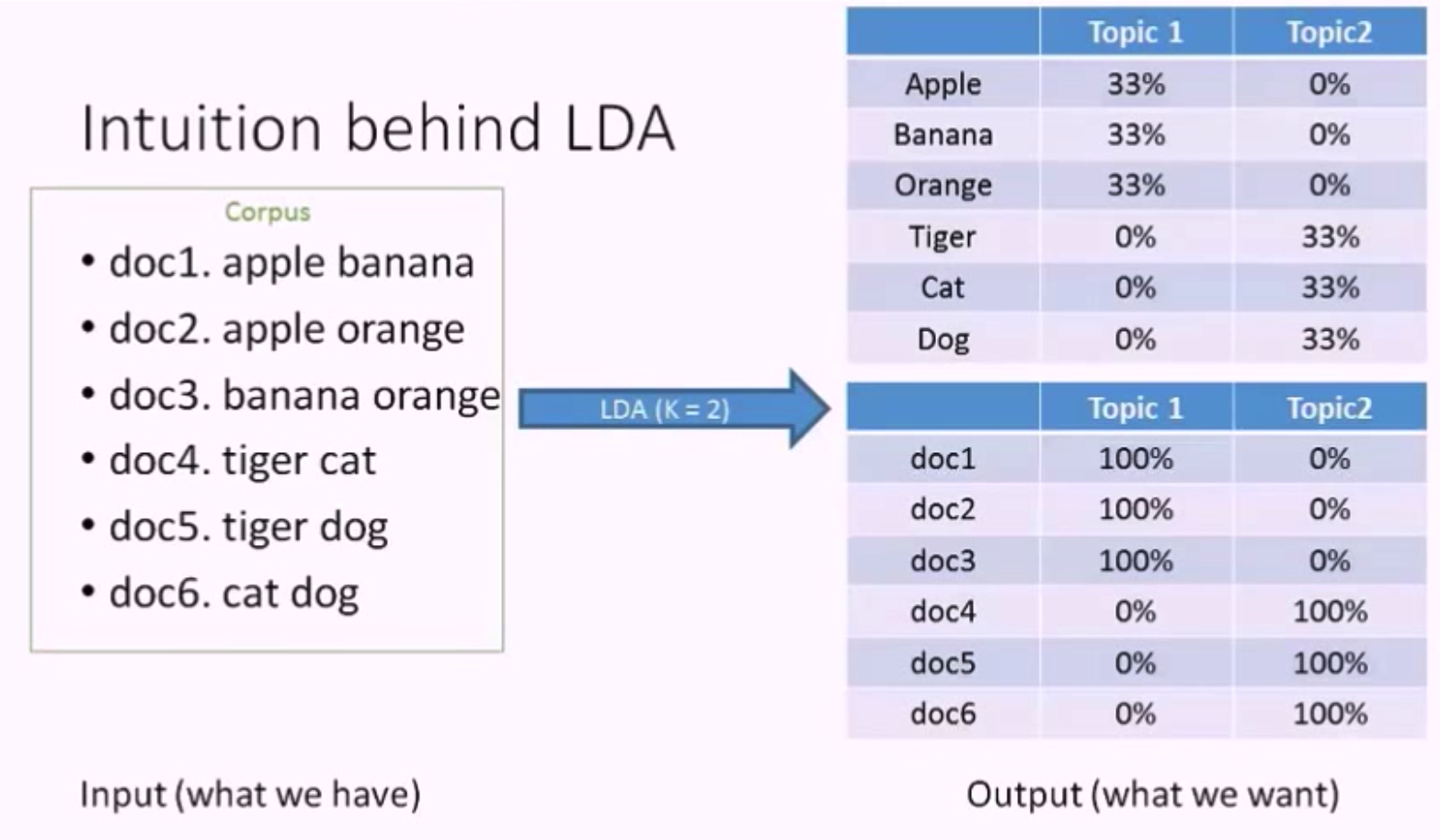LDA 的第一个输出是主题的单词分布 (Topic - Word Distribution)，由上图可知，主题1的单词概率分布是 apple banana orange 各占 33%，这就是 LDA 描述主题的方法，她使用单词 (Word / Term) 来描述主题。

LDA 的第二个输出是文档的主题分布 (Doc - Topic Distribution)，由上图可知，文档1中单词 apple banana 都是关于水果的，所以它的主题是 100% 关于主题1 (水果)，文档4的主题是 100% 关于主题2 (动物)。当然，一个文档中是可以包含多个主题的，此处的例子只包含一个主题是因为文档中单词比较少，正好都关于一个主题。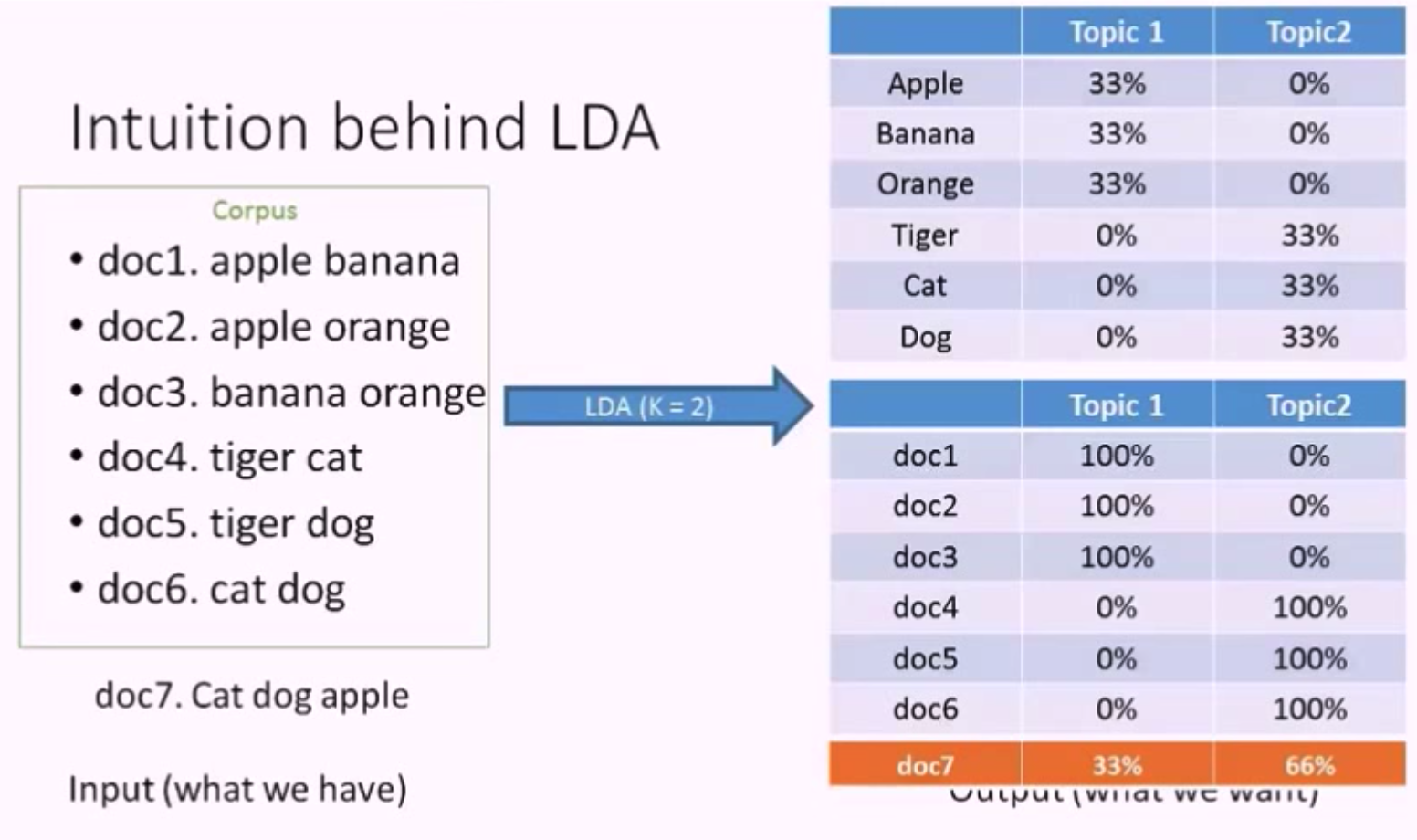## 贝叶斯定理 Bayes Theorem

• 概率: 用于在已知一些参数的情况下，预测接下来的观测所得到的结果。
• 似然: 用于在已知某些观测所得到的结果时，对有关事物的性质的参数进行估计。
• $P(A|B)$: 是已知 B 发生后 A 的条件概率，也由于得自B的取值而被称作 A 的后验概率。
• $P(A)$: 是 A 的先验概率。之所以称为”先验”是因为它不考虑任何 B 方面的因素。
• $P(B|A)$: 是已知 A 发生后 B 的条件概率，也由于得自A的取值而被称作 B 的后验概率。
• $P(B)$: 是 B 的先验概率。
• $\frac{P(B|A)}{P(B)}$: 称作标准似然 (standardised likelihood)

LDA 是基于贝叶斯模型的，她是一个三层贝叶斯模型。

## 伽玛函数 Gamma Function

Gamma 函数可以看成是阶乘在实数集上的延拓：

LDA 所涉及的概率分布中会用到 Gamma 函数以及它的性质，所以先介绍一下 Gamma 函数。

## 一些概率论 Some Probability Theory

LDA 主题模型中涉及了许多概率分布，列举如下。

### 贝塔分布 Beta Distribution

Beta分布的定义：对于参数 $\alpha > 0, \beta > 0$ , 取值范围为 [0, 1] 的随机变量 X 的概率密度函数为：

## 隐含狄利克雷分布主题模型 LDA Topic Model

### 使用场景 Use Cases

• 自动推测出文档集中讨论的主题
• 推测出的主题信息可以用于总结和组织文档，也可以用于降低特征 (featurization) 和维度 (dimensionality)
• 文档 X 在讨论什么？-> 主题识别
• 文档 X 和 文档 Y 的相似度是多少？-> 文本相似度计算
• 如果我对主题 Z 感兴趣，那么哪篇文档最对我的胃口？ -> 推荐系统
• 其他使用场景
• 情感分析 Sentiment analysis
• 图片目标定位 Object localization for images
• 音乐自动谐波分析 Automatic harmonic analysis for music
• 生物信息学 Bioinformatics

### 假设 Assumptions

• Documents with similar topics will use similar groups of words
• Document definitions / modeling:
• Documents are probability distributions over latent topics 文档是潜在主题的概率分布
• Topics are probability distributions over words 主题是单词的概率分布

Doc - Topic Distribution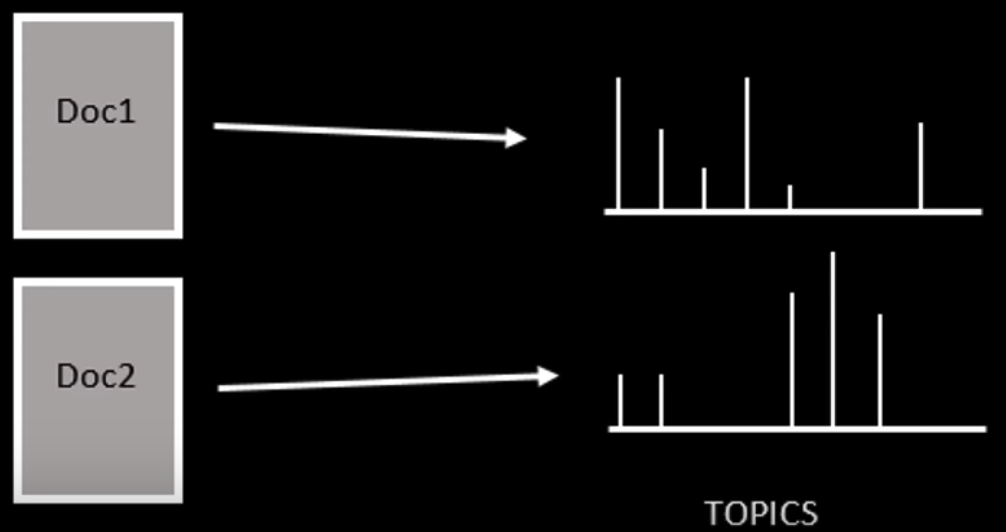Topic - Word Distribution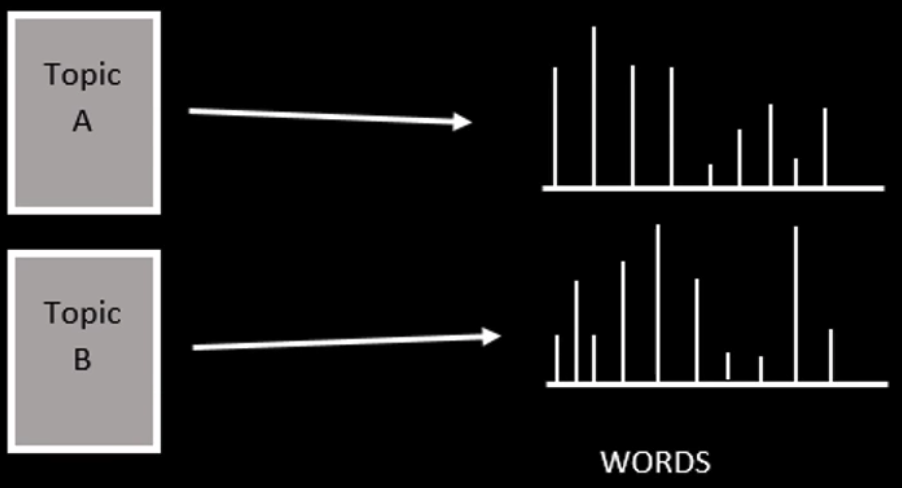LDA 认为单词携带了强烈的语义信息，包含相似主题的文档中会出现相似的单词组。

LDA 模型与别的词袋模型的不同之处就在于，它利用了单词的概率分布而不是单词的出现频率。其他词袋模型可能会考虑文档中出现得最频繁的单词，而 LDA 使用了一种更全面的方法：考虑主题的单词分布。

### 盘子表示法 Plate Notation

LDA 是一个三层贝叶斯模型，如下图所示，矩形框是“盘子”，代表复制品 (replicates)。

• 大盘子代表文档层
• 中盘子代表单词层
• 小盘子代表主题层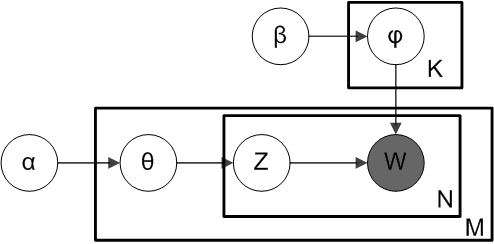• $M$: 语料库中文档的数量
• $N$: 文档中单词的数量
• $K$: 语料库中主题的数量
• $\alpha$: 每篇文档的主题分布 (先验狄利克雷分布) 的参数，是一个 K 维向量，K 是主题数量
• 越高，代表每篇文档可能包含更多的主题，而不只是包含一个或者两个特定的主题
• 越低，代表每篇文档包含的主题数越少
• $\theta_i$: 文档 $i$ 的主题分布
• $z_{ij}$: 文档 $i$ 中第 $j$ 个单词的主题编号，服从多项式分布
• $\beta$: 每个主题的单词分布 (先验狄利克雷分布) 的参数，是一个 V 维向量，V 是文档中单词的数量
• 越高，代表每个主题可能包含更多的单词
• 越低，代表每个主题包含的单词数越少
• $\varphi{z{ij}}$: 主题 $z_{ij}$ 的单词分布
• $w_{ij}$: 特定的单词，服从多项式分布

### 生成过程 Generative Process

LDA 认为一篇新文档是以这样的方式生成的：

• For each document $i$, draw $\theta_i \sim Dirichlet(\alpha), d = 1…D$
• For each word $j$ in document $i$:
• Sample from $\thetai$ , draw a topic index $z{ij} \sim Multinomial(\theta_i)$
• Sample from $\varphi{z{ij}}$ , draw the observed word $w{ij} \sim Multinomial(\varphi{z_{ij}})$

For parameter estimation, the posterior distribution is:

• 决定文档中单词的数量
• 给文档选择一个主题分布 (i.e. 20% topic A, 30% topic B, 50% topic C)
• 生成文档中的单词，通过：
• 首先基于文档的主题分布选一个主题
• 再基于主题的单词分布随机选一个单词
• 重复上面两步，直到单词数量达到一开始设定的上限

• 设定文档的长度为 1000 个词
• 假设文档的主题分布是：30% Arts, 20% Budgets, 30% Children, 20% Education
• 首先基于文档的主题分布选一个主题 (根据我们的主题分布，1000 个词中大约 300 词来自主题 Arts)
• 再基于主题的单词分布随机选一个单词
• 重复上面两步 1000 次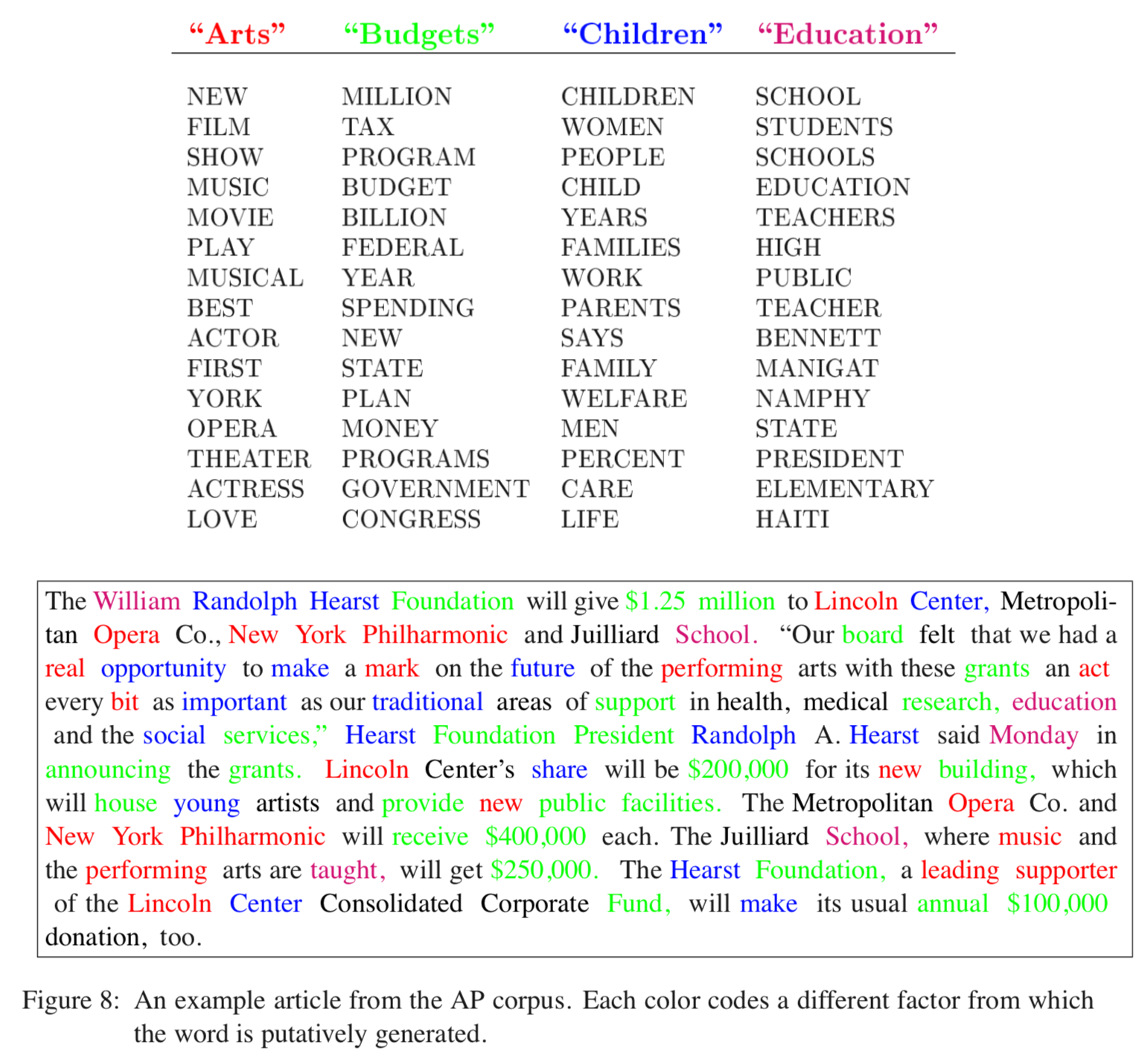LDA 求解文档的主题分布实际上是上述生成过程的逆过程，从文档开始，反过来去发现文档中的主题。

• 生成过程：主题 -> 文档
• 逆过程：文档 -> 主题

### 逆过程 Working Backwards

LDA 从文档层回溯，去推测哪些主题可能用于生成该文档。

• 随机给每篇文档中的每个单词分配 (assign) k 个主题中的一个主题
• 对于每篇文档 d，更新 (重新采样 Resample) 单词的主题
• 假设除了当前文档之外的所有主题分配是正确的
• 计算两个概率
• 当前文档 d 中的单词被分配到主题 t 的概率 P(topic t | doc d)
• 所有文档中被分配到主题 t 的单词中是单词 w 的概率 P(word w | topic t)
• 基于这两个概率的乘积 P(topic t | doc d) * P(word w | topic t) 分配给单词 w 一个新的主题
• 不断重复上述步骤，最终达到稳定状态，这时可以认为所有主题的分配是合理的，得到文档的主题分布

### 解法 Existing Solutions

• Variational EM (Expectation Maximization)
• Gibss Sampling

Online LDA Algorithm:

• is streamed: training documents may come in sequentially, no random access required.
• runs in constant memory w.r.t. the number of documents: size of the training corpus does not affect memory footprint, can process corpora larger than RAM, and
• is distributed: makes use of a cluster of machines, if available, to speed up model estimation.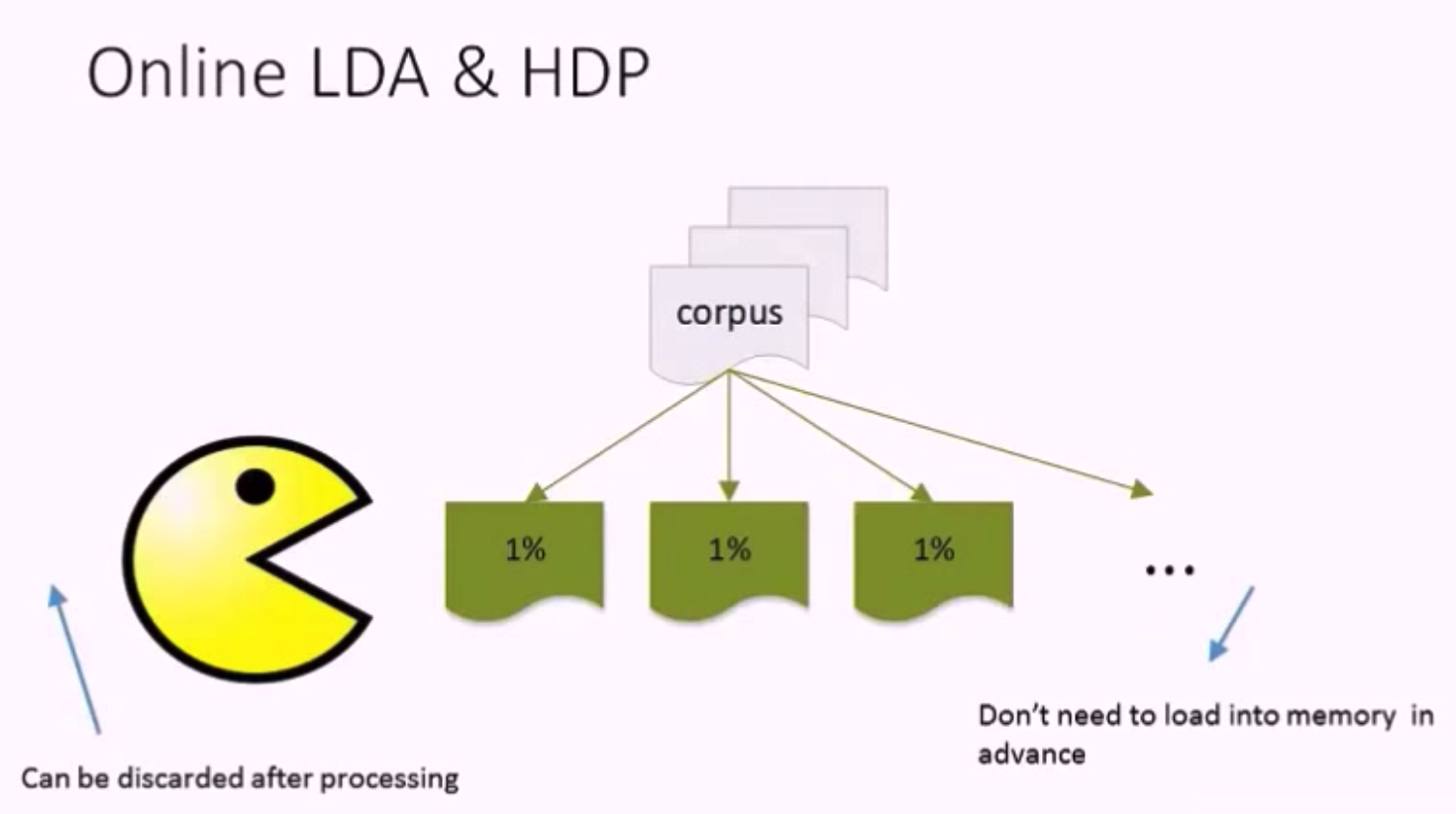Yee Whye Teh 等人提出的层次狄利克雷过程 (Hierarchical Dirichlet Processes) 可以自动地确定 topic 的个数，也可以用于参考。

## 吉布斯采样算法 Gibss Sampling Algorithm

• 首先对所有文档中的所有词遍历一遍，为其都随机分配一个主题，即 $z_{m,n} = k \sim Mult(1/K)$ ,其中 $m$ 表示第 $m$ 篇文档，$n$ 表示文档中的第 $n$ 个词，$k$ 表示主题，$K$ 表示主题的总数，之后将对应的 $n^{(k)}_m+1, n_m+1, n^{(t)}_k+1, n_k+1$, 他们分别表示在 $m$ 文档中 $k$ 主题出现的次数，$m$ 文档中主题数量的和，$k$ 主题对应的 $t$ 词的次数，$k$ 主题对应的总词数。
• 之后对下述操作进行重复迭代。
• 对所有文档中的所有词进行遍历，假如当前文档 $m$ 的词 $t$ 对应主题为 $k$，则 $n^{(k)}_m-1, n_m-1, n^{(t)}_k-1, n_k-1$, 即先拿出当前词，之后根据 LDA 中 topic sample 的概率分布 sample 出新的主题，在对应的 $n^{(k)}_m, n_m, n^{(t)}_k, n_k$ 上分别 +1。

## 结论 Conclusions

LDA 接受的输入是若干文档。她认为每篇文档中的单词是语义相关的。她试图想明白每篇文档是如何创造出来的。我们只需要告诉她有多少个主题需要构造，她就会根据主题的数量在语料库上生成主题和单词的分布。基于她的输出，可以预测新文档的主题分布，计算文档之间的相似度，文本分类。

LDA

• Input
• 文档集 (Corpus)
• K: the number of topics
• Output
• 主题的单词分布 (Topic - Word Distribution) -> 每个主题在讨论什么
• 文档的主题分布 (Doc - Topic Distribution) -> 每篇文档中包含了哪些主题

• 对于每一个主题均可找出一些词语来描述它
• 是一个有效的主题建模工具
• 在许多领域已经展示出了很好的结果，比如推荐，文本分类

• 必须事先指定主题的数量 K，而这个 K 的选取是困难的，需要大量的试验来确定
• 假如语料库中有许多关联的主题，狄利克雷主题分布无法捕捉主题间的相关性